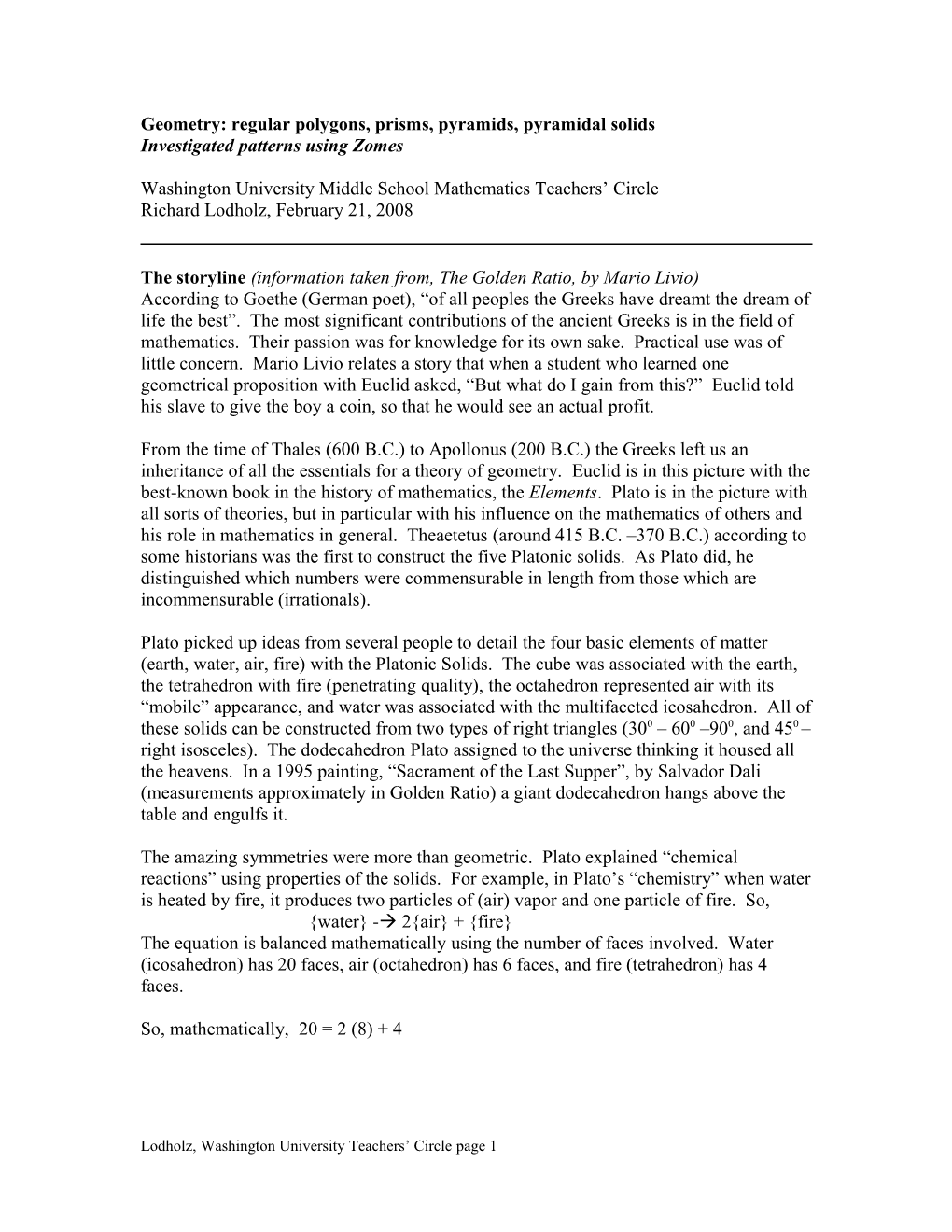# Mathematics: Ideas Created from PatternsGeometry: regular polygons, prisms, pyramids, pyramidal solids

Investigated patterns using Zomes

Washington University Middle School Mathematics Teachers’ Circle

Richard Lodholz, February 21, 2008

The storyline (information taken from, The Golden Ratio, by Mario Livio)

According to Goethe (German poet), “of all peoples the Greeks have dreamt the dream of life the best”. The most significant contributions of the ancient Greeks is in the field of mathematics. Their passion was for knowledge for its own sake. Practical use was of little concern. Mario Livio relates a story that when a student who learned one geometrical proposition with Euclid asked, “But what do I gain from this?” Euclid told his slave to give the boy a coin, so that he would see an actual profit.

From the time of Thales (600 B.C.) to Apollonus (200 B.C.) the Greeks left us an inheritance of all the essentials for a theory of geometry. Euclid is in this picture with the best-known book in the history of mathematics, the Elements. Plato is in the picture with all sorts of theories, but in particular with his influence on the mathematics of others and his role in mathematics in general. Theaetetus (around 415 B.C. –370 B.C.) according to some historians was the first to construct the five Platonic solids. As Plato did, he distinguished which numbers were commensurable in length from those which are incommensurable (irrationals).

Plato picked up ideas from several people to detail the four basic elements of matter (earth, water, air, fire) with the Platonic Solids. The cube was associated with the earth, the tetrahedron with fire (penetrating quality), the octahedron represented air with its “mobile” appearance, and water was associated with the multifaceted icosahedron. All of these solids can be constructed from two types of right triangles (300 – 600 –900, and 450 – right isosceles). The dodecahedron Plato assigned to the universe thinking it housed all the heavens. In a 1995 painting, “Sacrament of the Last Supper”, by Salvador Dali (measurements approximately in Golden Ratio) a giant dodecahedron hangs above the table and engulfs it.

The amazing symmetries were more than geometric. Plato explained “chemical reactions” using properties of the solids. For example, in Plato’s “chemistry” when water is heated by fire, it produces two particles of (air) vapor and one particle of fire. So,

{water} - 2{air} + {fire}

The equation is balanced mathematically using the number of faces involved. Water (icosahedron) has 20 faces, air (octahedron) has 6 faces, and fire (tetrahedron) has 4 faces.

So, mathematically, 20 = 2 (8) + 4

Geometry has two great treasures; one is the Theorem of Pythagoras; the other, the division of a line into extreme and mean ratio. The first we may compare to a measure of gold; the second we may name a precious jewel.

Johannes Kepler (1571-1630)

Theorem of PythagorasGolden Ratio, fphi”

Recall the powerful geometric concepts for similar triangles. If two angles of one triangle equal two angles of another, the triangles are similar and corresponding sides are proportional.

Task 1.(Using treasure 1) Find formula for area of equilateral triangle with side s, formula for area of hexagon with side s.

Talk about concept of using central angle to side to find one area then multiply by the number of sides to find area of polygon

Task 2.(Using treasure 2) Find numeric value of f (mention, in a sense there are three segments … (a+b), a, and b.

Zomes are a great tool for seeing patterns in geometry.

Architect, Steve Baer, created the Zome System around 1970 to study the zone of the dome. The Zomes are comprised of three main sets of struts: blue, yellow, and red. Each set has three lengths, which represent the golden ratio (division of a line into extreme and mean ratio). We verified this by illustrating that:

(a) b2 + b1 = b3

and

(b) making two similar triangles, one with 2 b1’s and a b2

and the second with 2 b2’s and a b3

This gave us b3/b2 = b2/b1 , which is substituted in (b2 + b1)/b1 = b2/b1

We illustrated that x1 + x2 = x3 held for each color by building two other similar triangles: one with b2, y2 and r3 , and the second with b1, y1 and r2

This produced:b2/b1 = y2/y1 = r3/r2

Constructions:

Regular triangles, squares, pentagons, hexagons(with blue struts)

Diagonals of square(green struts)

Cubes

Tetrahedron in the cube

Trihedral angle of cube with face diagonals and diagonal of cube to illustrate the concept of volume of pyramid equals 1/3 volume of related prism.

Prisms using the hexagonal base, concept of volume equal area of base times height.

Pyramids using the other three polygonal bases constructed.

Pentagonal pyramid reflection horizontally through vertex. Put in vertical axis with red struts, spin to simulate cone.

Dodecahedron

Triangular pyramid reflection horizontally through vertex.

Icosahedron

Dodecahedron without central struts.

Icosahedron without central struts.

Lodholz, Washington University Teachers’ Circlepage 1